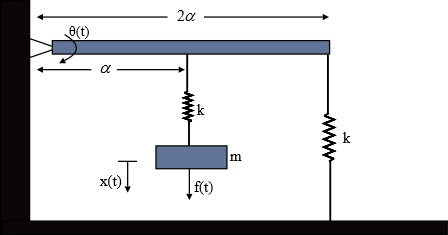# Consider the mechanical suspension system show below. The beam of length 2alpha is initially...

## Question:

Consider the mechanical suspension system show below. The beam of length {eq}2\alpha {/eq} is initially horizontal at its equilibrium position. A force f(t) is applied to the mass m as shown in the figure. Assuming small displacements, write the linearized dynamic equations that describe the motion of the system with respect to the system parameters m, k, {eq}\alpha {/eq} and J (where J is the moment of inertia of the beam).## Spring

Spring is a helical structure made up of elastic material, which has high stiffness. Spring used as a damper on the automobiles to absorb shocks during the travelling. The restoring force that a spring imparts on any object is termed as a spring force.

## Answer and Explanation:

Given Data:

• The length of the beam is: {eq}{L_b} = 2\alpha {/eq}
• The Force applied to the mass is: {eq}f\left( t \right) {/eq}
• The mass of the block is: {eq}{m_b} = m {/eq}
• The spring constant is: {eq}k {/eq}
• The moment of inertia of the beam is: {eq}{\rm{J}} {/eq}

The expression for the torque due to the spring without mass at hinge,

{eq}\begin{align*} {T_{wm}} &= {F_{wm}} \times {d_{wm}}\\ &= k\left( {{L_b}} \right)\left( {{L_b}\theta } \right) \end{align*} {/eq}

Here {eq}{F_{wm}} {/eq} is the resisting force of the spring and {eq}{d_{wm}} {/eq}is the distance of the spring from the hinge.

Substitute the given data in the above equation,

{eq}{T_{wm}} = k{\left( {2\alpha } \right)^2}\theta {/eq}

The expression for the torque for the spring with mass at hinge,

{eq}\begin{align*} {T_m} &= {F_m}{d_m}\\ &= k\left( {\dfrac{{{L_b}}}{2}\theta } \right)\left( {\dfrac{{{L_b}}}{2}} \right) \end{align*} {/eq}

Here {eq}{F_m} {/eq} is the resisting force of the spring and {eq}{d_m} {/eq}is the distance of the spring from the hinge.

Substitute the given data in the above equation,

{eq}\begin{align*} {T_m} &= k{\left( {\dfrac{{2\alpha }}{2}} \right)^2}\theta \\ &= k\theta {\alpha ^2} \end{align*} {/eq}

The expression for the torque for mass at hinge,

{eq}{T_{fm}} = {m_b}g{d_m} {/eq}

Substitute the given data in above expression,

{eq}{T_{fm}} = mg\left( {\alpha \theta } \right) {/eq}

The expression for the torque due to moment of inertia at hinge

{eq}{T_j} = J\ddot \theta {/eq}

The expression for the dynamic equation of motion,

{eq}\begin{align*} {T_J} + {T_{wm}} + {T_m} + {T_{fm}} &= F\left( t \right)\\ \left( {J\ddot \theta } \right) + \left( {mg\left( {\alpha \theta } \right)} \right) + \left( {k\theta {\alpha ^2}} \right) + \left( {k{{\left( {2\alpha } \right)}^2}\theta } \right) &= F\left( t \right)\\ J\ddot \theta + \left( {mg\alpha + 5k{\alpha ^2}} \right)\theta &= F\left( t \right) \end{align*} {/eq}

Thus the expression for the equation of motion of the given system is {eq}F\left( t \right) = J\ddot \theta + \left( {mg\alpha + 5k{\alpha ^2}} \right)\theta {/eq}

#### Learn more about this topic:Torque: Concept, Equation & Example

from UExcel Physics: Study Guide & Test Prep

Chapter 7 / Lesson 4
19K